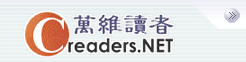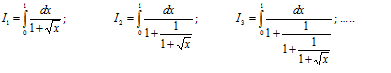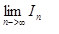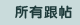设万维读者为首页广告服务技术服务联系我们关于万维简体繁体手机版
 首页 新闻 论坛 博客 视频 分类广告 购物版主：
 五 味 斋 茗香茶语 天下论坛 竞技沙龙 彩虹之约 摄友部落 诗词歌赋 七荤八素 高山流水 海 二 代 教育学术 笑林之声 健康生活 史地人物 军事天地 跨国婚姻 恋恋风尘 灵机一动 股市财经 加国移民 流行前线 新 大 陆 影视娱乐 焦点房谈 我爱我车 美国移民
 万维读者网 > 灵机一动 > 帖子
 积分智力题试解 （修改） 送交者: 粱远声 2017年08月11日16:35:12 于 [灵机一动] 发送悄悄话 定义：求积分智力题试解先讨论被积函数的分母。f1(x) = 1 + x^0.5,   f2(x) = 1 + 1/f1(x),  f3(x) = 1 + 1/f2(x),fn+1(x) = 1 + 1/fn(x)fn(x) > 1fn+1(x) - fn-1(x) = 1 + 1/(1+1/fn-1(x)) - fn-1(x) = (1 - fn-1(x) - fn-1^2(x))/(fn-1(x) + 1) < 0这样我们得到fn+1(x) < fn-1(x)                        (1)fn(x) > 1                                     (2)(1) 说明x在（0，1) 区间奇数列单调减少，偶数列单调减少。（2）说明数列有下界。所以奇数列收敛，偶数列收敛。接下来看看数列的极限是什么。在（0，1) 区间任取x0, 假设奇数列收敛于a, 根据递推公式fn+1(x0) = 1 + 1/(1+1/fn-1(x0))           (3)得到a = 1 + 1/(1+1/a)                                    (4)解得a = (1+5^0.5)/2                                      (5)(3)也是偶数列的递推公式，所以(5) 也是偶数列的极限值。奇偶列收敛于同一个极限，推出 fn(x0) 收敛。由于x0的任意性，fn(x) 收敛于常数 a。所以  In 收敛于 1/a
 0%(0)
 0%(0).ccc{width:675px;display:block;white-space:nowrap;overflow:hidden;float:left;-o-text-overflow: ellipsis;text-overflow:ellipsis;}实用资讯
 一周点击热帖 更多>>
 一周回复热帖